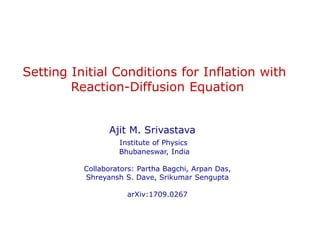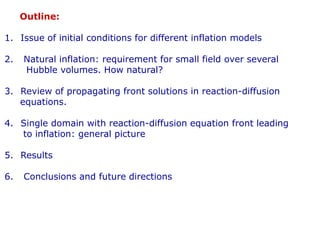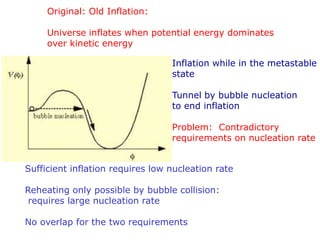Ce diaporama a bien été signalé.
Le téléchargement de votre SlideShare est en cours. ×
Publicité
Publicité
Publicité
Publicité
Publicité
Publicité
Publicité
Publicité
Publicité
Publicité
PublicitéChargement dans…3
×

## Consultez-les par la suite

1 sur 27 Publicité

# inflation.ppt

Inflation

Inflation

Publicité
Publicité

### inflation.ppt

1. 1. Setting Initial Conditions for Inflation with Reaction-Diffusion Equation Ajit M. Srivastava Institute of Physics Bhubaneswar, India Collaborators: Partha Bagchi, Arpan Das, Shreyansh S. Dave, Srikumar Sengupta arXiv:1709.0267
2. 2. Outline: 1. Issue of initial conditions for different inflation models 2. Natural inflation: requirement for small field over several Hubble volumes. How natural? 3. Review of propagating front solutions in reaction-diffusion equations. 4. Single domain with reaction-diffusion equation front leading to inflation: general picture 5. Results 6. Conclusions and future directions
3. 3. Original: Old Inflation: Universe inflates when potential energy dominates over kinetic energy Inflation while in the metastable state Tunnel by bubble nucleation to end inflation Problem: Contradictory requirements on nucleation rate Sufficient inflation requires low nucleation rate Reheating only possible by bubble collision: requires large nucleation rate No overlap for the two requirements
4. 4. New Inflation 0 ) ( 3           V H Field equation: Inflate during slow roll down Reheat: roll down the slope and oscillate Question: Why should field start from very small values? Note: this potential corresponds to a second order phase transition. Note: the field always sits at the minimum: continuous change of vev for 2nd order transition.
5. 5. New Inflation Thus: Impossible to argue for small initial field amplitude for this (second order transition) effective potential. Need: metastable vacuum with small barrier: Field initially localized at 0 (after phase transition), tunnels through the barrier. Then rolls slowly for inflation Universe consists of different Inflating bubbles.
6. 6. Important point about initial condition for these two models of inflation: Required value of field (close to zero) naturally set by initially thermal equilibrium stage with restoration of symmetry. After the transition,  settles at   0 due to the presence of metastable vacuum. So,   0 does not require any fine tuning. For other models, chaotic, natural, initial value of the field is not set naturally in this manner. Rather, it is supposed to explore entire allowed (relevant) field values. Inflation occurs wherever the field has correct value. Important: Correct initial value always refers to the value in the entire Hubble volume. For randomly varying field one says that it is the field value averaged over the Hubble volume which should satisfy required initial conditions. This is an important statement: during initial stages of inflation this average value should change in the manner of “slow roll”.
7. 7. Chaotic inflation and Natural inflation: No fine tuning of Shape: Require large field amplitudes for slow roll. Very large roll down time (very flat potential shape), so large distance to travel (large field amplitude), with low gradient We consider natural Inflation as example: We will not discuss the issue of the viability of the models. Any model having inflaton field has to address the issue of initial conditions for the field. Also: our model can be extended to other models of inflation Potential for natural inflation: )] / cos( 1 [ ) ( 4 f V      Minimum at   p f: f ~ mpl ,  ~ 1015 GeV
8. 8. Note: This potential is exactly of the same shape as axion potential for QCD (and also for chiral sigma model in low energy effective theory of QCD): Two very different energy scales arise naturally. Note: Potential always tilted: Tilt is negligible at energy scales much larger than . Successful inflation requires field very close to saddle point Q, most importantly: over several Hubble volumes. True vacuum Veff T > TC T = 0 P GUT scale 4 f ~ mpl  Q
9. 9. Can one assume that the entire required value of the field spans the Hubble volume? May be more natural near the Planck scale as all relevant length scales (including the Hubble scale) are of same order. BUT: No reason to expect this when scale of inflation is below the Planck scale. Then all relevant correlation lengths are much smaller than the Hubble scale. So field must have large variations within the entire Hubble volume. One can say that the relevant thing is the average value of the field over the Hubble volume. Suppose average value of the field in the Hubble volume is 0.1. With  varying randomly over many correlation volumes (domains), this means that many such “domains” will have  larger than 0.1.  will roll down much faster in those domains. This means that the average value of  will increase faster than the roll down time calculated for the averaged  itself. So calculation not consistent.
10. 10.   p f )] / cos( 1 [ ) ( 4 f V      f ~ mpl  ~ 1015 GeV 4 Natural setting: Thermal initial conditions with T >  (but T much less than mpl). Non-zero probability for initial ~ 0 (recall, this is a saddle point). Take conservatively, initial ~ 0.1 (2pf), probability ~ 0.1 We need this to hold for at least several Hubble volumes. Then only FRW equations are relevant leading to vacuum energy dominance for inflation. Again, conservatively, take only one Hubble volume. Let us then assume that  has roughly the required initial value In the entire Hubble volume
11. 11. For radiation dominated era down to T ~  , we have: H ~ 1/t , and T ~ t-1/2 So: H-1 GUT = H-1 pl T2 pl/T2 GUT Take thermal correlation length x ~ T-1, then H-1 GUT/ xGUT = (H-1 pl/ xpl )Tpl/TGUT With all Planck scale quantities of order mpl, and TGUT ~ 1015 GeV, H-1 GUT/ xGUT = 104 So, each Hubble volume has about 1012 correlation volumes. Field not expected to be correlated beyond correlation length. So, each correlation volume should have independently varying field magnitude, especially with axionic almost flat potential. (Tilted potential makes it even harder to assume values close to The top of the potential).
12. 12. For each correlation volume, required value of  ~ 0.1 had probability 0.1 So, for the Hubble volume with 1012 correlation domains, the probability that  ~ 0.1 in the entire Hubble volume is (0.1)10^12 which is completely negligible. One may say that for lengths much larger than the correlation Length one should take the probability to be exp(-r/x), which Will not be that small. However, exp(-r/x) represents decay of correlation as an Expectation value, meaning on average. This will not mean field having precisely correlated value over The Hubble volume at each point. Anywhere the field deviates from required slow roll regime, It will roll down fast spoiling slow roll condition for the average Field value. Same argument holds for T = 0 case with T = 0 correlation length being the relevant scale (controlled by parameters of the theory).
13. 13. This is a serious problem in assuming a reasonable initial condition for inflation. One needs to specify the specific conditions under which the required initial conditions can arise in any inflation model. We argue that this can be achieved “naturally” by precisely invoking (rather than neglecting) the correlation domain structure of variation of field inside the Hubble volume. We will not require initial small (precise) value of the field over any extended region. It will be very small in a small region (smaller than the Hubble size) and will be assumed to smoothly change to large value (vev) over the domain size. In principle the domain size can even be as small as a single Correlation domain, it requires more detailed investigations Of allowed values of domain size.
14. 14. The issue of initial homogeneity requirement of inflaton has been addressed by Linde recently using numerical simulation of full Einstein field equations coupled to scalar inflaton field (arXiv: 1511.05143). He finds that for inflation one requires, not just the average value of the field, but also the fluctuation of the field in a Hubble region to remain within the slow roll region of the potential. Otherwise, the field quickly rolls down to minimum, being pulled down by the large field regions. As we mentioned, such homogeneous field is not a reasonable requirement from the picture of correlation domains where full range (allowed by the energy/temperature considerations) of random variations of the field occurs across the correlation region. In our model, the field is small only in a small region (field domain), and varies smoothly to large value (vev) over the domain size. Thus, even for one domain the fluctuation (variation) of the field lies outside the slow roll region.
15. 15. We use specific features of the reaction-diffusion equation to address this issue Reaction-Diffusion equations : Quick review Diffusion equation: In 1-d it has solution of the form: Note: Diffusion equation has no traveling wave solution of the form u(x-vt). Modify the equation by introducing “reaction term” f(u). (used e.g. in the context of biological systems, where the reaction term represents interaction of species). This is the “Reaction-Diffuion equation. It has traveling wave (and static) solutions, with appropriate boundary conditions. Compare with the field equations: In the high dissipation limit, this is same as the reaction-diffusion Eqn u D t u 2     Dt x e Dt u t x u 4 / 2 / 1 0 2 ) 4 ( ) , ( -  p ) ( 2      V -    -    ) ( ) .( u f u D t u      
16. 16. Nontrivial field evolution with reaction-diffusion equation for specific boundary conditions: With this initial profile for  Expected evolution:  rolls down to vev = 0 Actual evolution Development of Well formed front which propagates with definite velocity Which can be 0 Take this potential Note: Non-zero vev (and a maximum) necessary for the propagating (or static) front. For the above potential field rolls down quickly 0
17. 17. Recall: potential for QCD axion case, or for QCD chiral sigma model: As mentioned earlier, Natural inflation has the same form of potential For QCD chiral sigma model, we had studied evolution of a field profile interpolating between points Q and P (along the tilted circle) with dissipative field equations. These equations became exactly the same as specific reaction-diffusion equation known as the Newell-Whitehead equation. One would expect field at point Q to quickly roll down to P. However: well defined front forms and moves inwards, just as the interface moves for a first order phase transition. Note: no metastable vacuum here, so no first order transition interface True vacuum Veff T > TC T = 0 P Q
18. 18. Lessons to learn from this: Note: the velocity of the front depends on the specific profile (different solutions have different velocities). There are also static fronts. So, in an expanding system (expanding plasma as for heavy-ion collisions, or expanding universe), expansion may dominate over slow shrinking of domain. In such a case, domain will actually expand, being stretched by the expansion, even though energetically it should have shrunk. For QCD case, we showed that this leads to formation of large DCC domains (disoriented chiral condensate domains). Its implications for expanding universe are then obvious:
19. 19. True vacuum Veff T > TC T = 0 P Q For expanding universe: think of this QCD potential as that for natural inflation. Think of the chiral field domain as domain for inflaton (axion) field, with zero of the field being at point Q (large vacuum energy). As the domain shrinks slowly (by inward motion of the wall), or it remains static, it gets stretched by the universe expansion. If expansion dominates, the domain will become larger, and eventually will dominate the energy in the Hubble volume. When vacuum energy starts dominating, universe will inflate. After the front exits the horizon, inflation will be established. Small value away from point Q will decide eventual roll down of the field and end of inflationary stage.
20. 20. Natural inflation with reaction-diffusion equation: Potential: )] / cos( 1 [ ) ( 4 f V      We take f = mpl ,   1015 GeV 0 ) ( 3 2 2      -        V H a Field equations for the inflaton: Scale factor evolution: Field energy: Radiation energy: (starting at GUT scale) Field profile taken as Tanh (for planar 1-d solution as well as for spherical 3-d solution) interpolating between the minimum of V at   p mpl and a point close to the Maximum of V with   e. Our results very insensitive to the initial profile. Even linear segments evolve into smooth profile and lead to inflation. 2 / 1 )] ( 3 8 [ radiation G a a H   p      ) ( 2 ) ( 2 1 2 2 2     V a      4 ~ - a radiation 
21. 21. Results: Profile of  (in units of f) for the initial field domain. The diameter of the domain is about 30 (in units of -1 ). HGUT -1 ~ 723 for GUT scale of 1015 GeV. This entire region is shown in the figure to illustrate that the field domain is much smaller initially than the causal horizon. However, the field domain has very large contributions from the gradient of the field due to non-trivial spatial profile of . Including this contribution for the region containing the field domain, the actual value of H-1 ~ 33, which is still larger than the size of the field domain.
22. 22. Black curve shows final field profile (in comoving coordinates) at the end of evolution, Blue curve shows initial profile. Very little change in the field profile (but huge expansion in physical coordinates, as seen in next figures)
23. 23. Evolution of different components of energy density. The vacuum energy becomes most dominant component at t ~ 14923. Time in units of -1 Evolution of H: power law, eventually turning over to turning over to a constant value of H signalling the beginning of the inflationary phase. This happens around at t ~15000 which coincides with the stage of the dominance of the vacuum energy in the figure above
24. 24. Black curve shows the location of the domain wall in physical coordinates, by noting down the position of the wall where  = 0.25 vev. Blue curve shows the value of H-1. (a)During initial stages, field domain expansion is insignificant compared to the increase in the value of H-1. (b) Towards the end of simulation, H-1 is practically constant while physical size of the domain (due to expansion of the Universe) keeps increasing. By the end of simulation here, at t ~10600, one can see that the domain exits the horizon, marking beginning of proper inflationary stage.
25. 25. Ending inflation: The evolution of Field value at the center of the domain (z=0) Profile near center: note it has smooth variation From this plot, one can make a rough estimate of time after which the field will become significantly non-zero, ending the period of inflation. A rough estimate will suggest that after time of order t ~ 1036, (z = 0) will become of order 1. This period of inflation crucially depends on the size z0 in the  profile. For a larger value of z0 duration of inflation will be larger, though at the same time it will become harder to justify a very large value of z0.
26. 26. Main points about the results: We show that one does not need to assume finely tuned value of the field in the entire Hubble volume for inflation. A single domain, much smaller than the Hubble volume, with field varying smoothly across the domain, can lead to Inflation. This occurs because of special reaction-diffusion equation solutions of dissipation dominated field equations. They lead to slowly moving (or static) fronts, instead of rapidly rolling field. Such smooth field profiles expected with general picture of correlation domains. Value close to the top of the potential (saddle point) taken only at one point, then it smoothly changes to the vacuum Value across the domain. Any Hubble volume with even one such domain will have Inflation.
27. 27. Future directions: Existence of slowly moving propagating front (instead of rapidly rolling field) is a general consequence of reaction-diffusion equations. This general picture can be applied to other models of inflation (which have potentials of correct types). Warm inflation has extra dissipation, this should lead to closer correspondence with the Reaction-diffusion equation. Effect of fluctuations etc. has to be studied, especially on the propagating front profile and its velocity. Then one must study Generation of inflationary fluctuations in this scenario. For Reaction-diffusion equations: For different reaction terms one gets different specific equations with well defined solutions. (We have shown that, with chiral sigma model potential one gets the Newell-Whithead equation, while for Polyakov loop potential, one gets the Fitzhugh-Nagumo equation used in population genetics). What will be the properties of the reaction-diffusion equation with cos() interaction term (as for the Natural inflation case)?